## Floatation Class 9 Important Questions and Answers Science Chapter 16

Based on Thrust and Pressure

Question 1.
Define ‘thrust’. (CBSE 2011, 2014, 2016)
The total force exerted by a body perpendicular to the surface is known as thrust.

More Resources

Question 2.
What is the unit of thrust in SI ? (CBSE 2013, 2016)
S.I. unit of thrust is newton (N).

Question 3.
Define the term ‘pressure’. (CBSE 2011, 2013, 2014, 2016)
Pressure is defined as the force acting perpendicular on unit area of the surface.

Question 4.
Name the SI unit of pressure. (CBSE 2013, 2016)
S.I. unit of pressure is N/m2 or pascal.

Question 5.
State the relationship between thrust and pressure. (CBSE 2010, Term I, 2013, 2014)
Thrust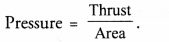Question 6.
Define 1 pascal. (CBSE 2014, 2015, 2016)
1 pascal is defined as the pressure exerted by 1 N force acting perpendicular on the surface of 1 m2 area.

Based on Buoyant Force

Question 7.
Define upthrust or buoyant force. (CBSE 2010, 2011, 2012, 2016)
The upward force exerted by a liquid on a body which is immersed in the liquid is known as upthrust or buoyant force.

Question 8.
State the factors on which upthrust or buoyant force depends. (CBSE 2010, 2011, 2012, 2016)

1. Size or volume of body immersed in a liquid,
2. Density of the liquid in which the body is immersed and
3. Acceleration due to gravity at the given place.

Question 9.
When an object is immersed in a fluid, name the two forces acting on it. (CBSE 2011, 2012, 2016)

1. Weight of object in the vertically downward direction,
2. Upthrust or buoyant force in the vertically upward direction.

Question 10.
Name the force experienced by an object in a fluid when immersed in it. What is its direction ?
(CBSE 2011)
Upthrust or Buoyant force. It acts on the object in upward direction.

Question 11.
Why does a block of wood held under water rise to the surface when released ? (CBSE 2011)
This is because the buoyant force or upthrust acting on the wooden block due to water is greater than the weight of the block.

Question 12.
An object of weight 200 N is floating in a liquid. What is the magnitude of the buoyant force acting on it? (CBSE 2011)
Magnitude of buoyant force = 200 N.

Question 13.
Explain the factors which determine whether an object floats or sinks when placed on the surface of water.
(CBSE 2011)

1. Volume of the object and
2. density of the water.

Question 14.
Name the force acting on a body, when it is fully or partially immersed in a liquid. (CBSE 2011)
Buoyant force.

Question 15.
What will be the direction of buoyant force when suppose the weight of an object is acting from north to south in the fluid ? (CBSE 2013)
From south to north as buoyant force acts opposite to the direction of the weight of the object.

Question 16.
A body of weight 20 N floats half submerged in a liquid. What is the buoyant force on the body ?
20 N

Based on Archimedes’ Principle

Question 17.
State Archimedes’ principle. (CBSE 2010, 2011, 2012, 2015)
When a body is immersed partially or completely in a fluid (liquid or gas), it experiences an upthrust or buoyant force which is equal to the weight of the fluid displaced by the body. The weight of the body decreases due to the buoyant force acting on the body, when immersed in a fluid. In other words, a body loses its weight, when immersed completely or partially in a fluid. The loss of weight of a body in a fluid is equal to the upthrust or buoyant force.
The upthrust or buoyant force = weight of fluid displaced by a body = weight of body in air – weight of body in fluid.

Question 18.
Write the relationship between buoyant force acting on an object and weight of the liquid displaced by it. (CBSE 2010, 2012)
Buoyant force = weight of liquid displaced by the object.

Question 19.
State the physical expression relating weight of the body in the liquid, actual weight of the body in air and the weight of the liquid displaced by the body. (CBSE 2011, 2013)
Weight of a body in a liquid = actual weight of the body – weight of the liquid displaced by the body.

Question 20.
Give any two examples where Archimedes’ principle is applied . (CBSE 2011, 2012)

Or

List two applications of Archimedes’ principle. (CBSE 2012, 2016)
Archimedes’ principle is applied in designing

1. ships and submarines and
2. lactometer used for testing the purity of milk.

Question 21.
State the principle on which working of hydrometer is based. (CBSE 2011)
Archimedes’ principle:
A Greek scientist Archimedes conducted many experiments and concluded that when a body or an object is immersed partially or completely in a liquid or a gas (i.e., fluid), it experiences an upthrust or buoyant force. The upthrust or buoyant force is equal to the weight of the fluid displaced by the body. As a result of the buoyant force acting on an object, the weight of the object decreases, when immersed in a fluid. The finding of Archimedes is known as Archimedes’ principle.
Archimedes’ principle is used to design :

1. the ships and submarines
2. the hydrometers to find the densities of liquids
3. the lactometers to test the purity of milk.

Based on Density and Relative density

Question 22.
Define density. Give SI unit of density. (CBSE 2011, 2012, 2014, 2015)
Density of an object is defined as mass per unit volume of the object. That is, density = mass/volume .
S.I. unit of density is kg/m3 (kg m-3).

Question 23.
Define relative density of a substance. (CBSE 2011, 2012, 2013, 2014, 2015)
It is defined as the ratio of the density of the substance to the density of water.

Question 24.
If the relative density of a substance is less than 1, will it float or sink in water ? Support your answer.
(Density of water = 1000 kg/m3). (CBSE 2011)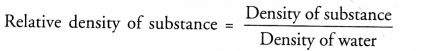As relative density of substance is less than 1, so density of substance is less than the density of water. Hence, the substance will float in water.

Question 25.
Relative density of aluminium is 2.7. Explain this statement. (Similar CBSE 2011)
Aluminium is 2.7 times heavier than the equal volume of water.

Question 26.
The density of a solid is 7.9 g cm-3 in air. What is the density of the solid in SI unit (kg m-3) ?
(CBSE 2016)
Density = 7.9 g cm-3 = 7.9 x 1000 kg m-3 = 7.9 x 103 kg m-3.

Question 27.
Relative density of gold is 19.3. The density of water is 1000 kg/m3. What is the density of gold in SI units ? (CBSE 2011, 2013, 2014, 2017)
Density of gold = Relative density of gold x density of water = 19.3 x 1000 kg/m3 = 19300 kg/m3

Based on thrust and pressure

Question 1.
Differentiate between thrust and pressure. State the unit in which they are measured.
Thrust: The total force exerted by a body perpendicular to the surface is known as thrust.
Pressure: Pressure is defined as the force acting perpendicular on unit area of the surface.
S.I. unit of thrust is newton (N).
S.I. unit of pressure is N/m2 or pascal.

Question 2.
The wheels of an army tank rest on a steel belt. Give reason.

Or

Why an army tank weighing more than a thousand tonne rests upon a continuous chain. (CBSE 2012, 2015)
Pressure exerted by army tank on the ground is given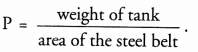Since area of steel belt is more than the area of the wheels of the tank, so tank exerts less force on the ground. Hence, the ground under the tank does not sink under the weight of the tank.

Question 3.
Why are railway tracks laid on large sized concrete sleepers ? Explain. (CBSE 2011)
The weight or thrust of the train is spread over large area of sleepers. Therefore, the pressure acting on the
ground under the sleepers is reduced. This prevents the sinking of the ground under the weight of the train.

Question 4.
Why do buildings have wide foundations ?
So that the weight of the buiding is spread over a large area and hence less pressure is exerted on the ground. Hence, the building is prevanted to sink under its weight.

Question 5.
Which will exert more pressure, 100 kg mass on 10 m3 or 50 kg mass on 4 m2 ? Give reason.
(CBSE 2011, 2014, 2015)Thus, P1 > P2.

Question 6.
Explain, why a truck or a motor bus has much wider tyres ? (CBSE 2011, 2015, 2016)
The weight of truck or motor bus spreads over large area on the surface of road or ground and hence less pressure is exerted by truck or a motor bus on the road. Therefore, the ground under them does not sink.

Question 7.
Account for the statement : “camel walks easily on sand but it is difficult for a man to walk on sand though a camel is much heavier than a man”. (CBSE 2011)
A camel walks easily on the sandy surface than a man inspite of the fact that a camel is much heavier than a man.
This is because the area of camel’s feet is large as compared to the area of man’s feet. So the pressure exerted by camel on the sandy surface is very small as compared to the pressure exerted by man. Due to large pressure, sand under the feet of a man yields (i.e., sink) and hence he cannot walk easily on the sandy surface. On the other hand, sand under the feet of camel does not sink much due to small pressure. Hence camel can walk and run easily on the sandy surface.Question 8.
A Sharp knife is more effective than a blunt knife. Why ? (CBSE 2012)

Or

Cutting and piercing tools are made sharp. Give reason. (CBSE 2015)
Pressure (P = F/A) exerted by sharp knife on an object is more than the pressure exerted by a blunt knife. Therefore, sharp knife is more effective in cutting the object than a blunt knife.

Question 9.
Why does an iron nail sinks in water but a wooden cork floats on water ? (CBSE 2011, 2012, 2015, 2016)
The weight of iron is greater than the upthrust of water on the iron nail, so iron nail sinks in water. On the other hand, the upthrust on cork is more than the weight of the cork. Hence wooden cork floats on water.

Question 10.
A balloon filled with hydrogen gas floats in air. Explain why ?
The upthrust or buoyant force acting on hydrogen balloon is greater than the weight of the hydrogen balloon. So it floats in air.

Question 11.
An object of volume V is immersed in a liquid of density p. Calculate the magnitude of buoyant force acting on the object due to liquid.
Magnitude of buoyant force acting on the object due to liquid = weight of liquid displaced
= mass of liquid displaced x g (∴ W = mg)
= Volume of body x density of liquid x g = Vρg
Thus, buoyant force depends upon

1. the volume of the immersed portion of the object and
2. density of the liquid.

Question 12.
An object is immersed in different liquids. Is same buoyant force acts on the object due to all liquids ?
(CBSE 2011)
We know, buoyant force acting on an object due to a liquid is directly proportional to the density of the liquid. Since different liquids have different densities, so different buoyant forces act on the object due to different liquids. An iron sphere is suspended with an iron string.

Question 13.
The length of the string increases (i.e. string is stretched). Now the iron sphere is completely immersed in water and the increase in length of the string decreases. Explain why? (CBSE 2011)
The length of the string increases due to the weight of the suspended iron sphere. When the sphere is immersed in water, buoyant force due to water acts on the sphere in the upward direction.
Now, the net force (or weight) acting on the string = actual weight of the sphere – buoyant force acting on it.
Since the net force acting on the string decreases and hence the extension of the string also decreases.

Question 14.
Two blocks, one of iron and other of wood are immersed in water at the same depth. Will both come upward ? Why ? (CBSE 2011)
Wooden block will come upward. This is because upthrust of water on the wooden block is greater than its weight. In other words, density of wood is less than the density of water and density of iron is greater than the density of water.

Question 15.
Lead has greater density than iron and both are denser than water. Is the buoyant force on a lead object greater than or lesser than or equal to the buoyant force on an iron object of the same volume ? Explain your answer giving reason. (CBSE 2011)
Buoyant force on an object depends upon

1. the volume of the object and
2. density of the liquid in which the object is immersed.

Since volume of both objects is same and both are immersed in water, therefore, buoyant force on lead object is equal to the buoyant force on iron object.

Question 16.
Explain, why a truck or a motor bus has much wider tyres ? (CBSE 2011, 2015, 2016)
The weight of truck or motor bus spreads over large area on the surface of road or ground and hence less
pressure is exerted by truck or a motor bus on the road. Therefore, the ground under them does not sink.

Question 17.
A ship is loaded in sea water to maximum capacity. What will happen if this ship is moved to river water ? Why? (CBSE 2011)
When ship is moved to river water from sea water, then upthrust acting on the ship decreases because density of river water is less than the density of sea water. Hence, the net weight of the ship will be more in river water than in sea water. Hence, more portion of the ship will be inside the river water.

Question 18.
Why do we feel lighter when we swim ?

Or

When we jump into a swimming pool, we feel lighter. Why ? (CBSE 2011)
When we swim in water, two forces acts on our body. One is the gravitational force equal to our weight in the downward direction and the other is the up thrust or buoyant force in the upward direction. Hence, the net downward force or the apparent weight of our body is less than our actual weight. Therefore, we feel lighter, when we swim.

Question 19.
An egg sinks in fresh water but floats in highly salty water. Give reason. (CBSE 2012)
Upthrust acting on egg in fresh water is less than the weight of egg. Therefore, it sinks in fresh water. However,
the density of salty water is much greater than the density of fresh water, so upthrust acting on egg in salty water is greater than the weight of egg. Therefore, it floats in salty water.

Question 20.
The following figure shows three identical blocks of wood floating in three different liquids A, B and C of densities d1, d2 and d3 respectively. Which of these has the highest density. Give reason to justify your answer.
(CBSE 2012)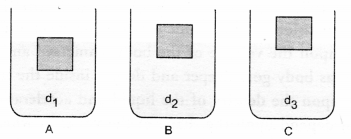In case of liquid C, upthrust is maximum. Since upthrust depends on the density of liquid, therefore, density of liquid C (i.e., is the highest.

Question 21.
When an object is immersed into the fluid, two forces act on the object in the vertically opposite directions. Name them and also write the factors on which the magnitude of these forces depends on. (CBSE 2012, 2015)

1. Weight of object = mg. It depends on the mass (or volume and density of object) and acceleration due to gravity.
2. Upthrust of fluid on the body = Vρg.
It depends on the volume (V) of immersed portion of object, density (ρ) of fluid and acceleration due to gravity.

Question 22.
The density of turpentine at 293 K is given as 870 kg m-3 Identify and write the names of substances that sink in turpentine at the same temperature.

 S.No. Substances Density (kg) 1 Wood 690 2 Ice 920 3 Rubber 970 4 Paraffin wax 900 5 Cork 240 6 Bone 1850

A substance sink in a liquid, if density of substance is greater than the density of liquid. Therefore, ice, rubber, paraffin wax and bone will sink in turpentine.

Question 23.
When a metallic block is immersed below the surface of a liquid, state and define the upward force acting on it. (CBSE 2014)
It is a buoyant force. The upward force exerted by a liquid is known as buoyant force. Buoyant force = Vρg, where V on the metallic block immersed in the liquid is the volume of metallic block immersed in the liquid and ρ is the density of the liquid.

Question 24.
State the condition under which an object floats on the surface of a liquid. What is the volume of the liquid displaced by the object ? (CBSE 2014, 2015)
An object floats on the surface of a liquid if upthrust on the body is greater than the weight of the object. Volume of the liquid displaced is equal to the volume of the body.

Question 25.
A cube of side 3 cm is immersed in water and then in saturated salt solution. In which case will it experience a greater buoyant force. If each side of the cube is reduced to 2 cm and then immersed in water, what will be the effect on the buoyant force experienced by the cube as compared to the first case for water. Give reason for each case. (CBSE 2015)
Initial volume of cube, Vi = (3 cm)3= 27 cm3
Final volume of cube, Vf = (2 cm)2 = 8 cm3

1. Buoyant force = Vρg, where p is the density of liquid. Since density of saturated salt solution is greater than the density of water, therefore, the cube will experience a greater buoyant force in saturated salt solution than in water.
2. Since the volume of the cube decreases, so the cube will experience a less buoyant force in water as compared to the first case.

Based on Archimedes’s Principle

Question 26.
While drawing water from a well, a bucket of water appears to be heavier as it comes out of the water.
Explain giving reasons. (CBSE 2011)

Or

Why is a bucket of water lighter when in water than when it is taken out of water ? (CBSE 2011)
When the bucket is inside water, its weight is less than its weight in air due to the upthrust of water.

Question 27.
A student took solid bodies of different shapes, sizes and materials and noted down the apparent loss in weight on partially or fully immersing the bodies in different liquids. Based on the observations, he wrote the following conclusions. Which conclusion is incorrect and why ?
(a) Upthrust depends upon the volume of the body immersed and density of liquid.
(b) Upthrust increases as body goes deeper and deeper inside the liquid.
(c) Upthrust depends upon the density of the liquid and acceleration due to gravity.
(d) Upthrust does not depend upon the shape of the vessel in which liquid is filled. (CBSE 2014)
Upthrust = Vρg, where V = volume of body immersed in liquid, ρ = density of liquid, g = acceleration due to gravity.
Therefore, conclusion (b) is incorrect as upthrust does not depend on the depth of the body inside the liquid.

Question 28.
If two equal weights of unequal volumes are balanced in air, what wil} happen when these are completely
dipped in water ? (CBSE 2011)
When two weights of unequal volumes are dipped in water, buoyant force acting on the weight of larger volume is more than that on the weight of smaller volume. Hence, weight of smaller volume in water is more than the weight of larger volume. Therefore, these weights are not balanced in water.

Question 29.
An object of mass 500 gm is immersed into a measuring jar containing water. The initial level of water in the measuring jar is 50 cc. Due to the immersion of the object, the water level in the measuring jar reaches to 100 cc. Calculate the mass of the water displaced due to the immersion of the object. (CBSE 2012)
Mass of water displaced = Volume of immersed portion of object x density of water
= (100 – 50) cm3 x 1 g cm-3 = 50 g.

Based on Density and Relative density

Question 30.
If a body is compressed to half its previous volume, what will be the effect on its density and why ?
(CBSE 2014)If volume of body becomes half its previous volume, then its density becomes double i.e., two times its previous value.

Question 1.
(a) State Pascal’s Law.
(b) Define upthrust or buoyant force. Describe an activity to show that a body loses its weight when immersed in a liquid.
(a) Pascal’s Law
A great French physicist and mathematician, Blaise Pascal gave a law regarding the pressure exerted by a fluid enclosed in a container. According to this Taw :
“Pressure applied to an enclosed fluid is transmitted undiminished to every portion of the fluid and the walls of the containing vessel This fact is shown in figure .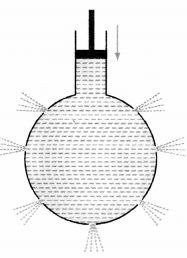The upward force exerted by a liquid on a body which is immersed in the liquid is known as the upthrust or buoyant force.
(b) Expression for Buoyant force or upthrust
Consider a cylindrical object of height h and area of cross-section A immersed completely in a liquid of density ρ (Figure 6).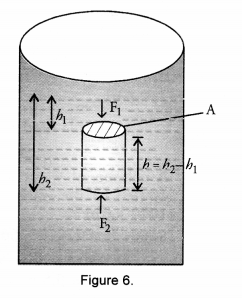Let h1 be the depth of the upper face of the object from the free surface of liquid. h2 be the depth of the lower face of the object from the free surface of the liquid.
The height of object, h = h2 – h1.
Volume of the object, V= Ah                                  ,
The pressure acting on the upper face of the object, P1 = h1ρg.
This pressure acts vertically downward on the upper face of the object.
Therefore, force acting on the upper face of the object in vertically downward direction,
F2 = P2A = h2Aρg                                                                   …(1)
The pressure acting on the lower face of the object in vertically upward direction, P2 = h2ρg
Therefore, force acting on the lower face of the object in vertically upward direction,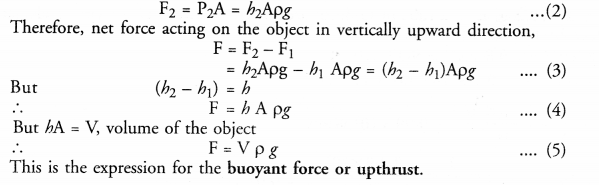Factors on which buoyant force or upthrust depends
Buoyant force is directly proportional to

1. the volume (V) of the object or body immersed in liquid
2. the density (ρ) of the liquid in which the object or body is immersed.
3. the acceleration due to gravity (g) at the given place.

Buoyancy: The tendency of an object to float in a liquid or the power of liquid to make an object float in it is called buoyancy.

Question 2.
Archimedes Principle has wide application in making ships and submarines. Explain. (CBSE 2013)